# R语言分布滞后线性和非线性模型（DLMs和DLNMs）分析时间序列数据

## 最近我们被客户要求撰写关于分布滞后非线性模型（DLNM）的研究报告。本文演示了在时间序列分析中应用分布滞后线性和非线性模型（DLMs和DLNMs）。

Gasparrini等人和Gasparrini阐述了DLMs和DLNMs的发展以及时间序列数据的实现。

# 数据

`````` date time year month doy dow death cvd resp temp dptp
1 1987-01-01 1 1987 1 1 Thursday 130 65 13 -0.2777778 31.500
2 1987-01-02 2 1987 1 2 Friday 150 73 14 0.5555556 29.875
3 1987-01-03 3 1987 1 3 Saturday 101 43 11 0.5555556 27.375
rhum pm10 o3
1 95.50 26.95607 4.376079
2 88.25 NA 4.929803
3 89.50 32.83869 3.751079``````

R语言中的分布滞后非线性模型（DLNM）与发病率，死亡率和空气污染示例

# 示例1：一个简单的DLM

``````cb(pm10, lag=15, argvar=list(fun="lin",
arglag=list(fun="poly",degree=4 ``````

`````` CROSSBASIS FUNCTIONS
observations: 5114
range: -3.049835 to 356.1768
lag period: 0 15
total df: 5
BASIS FOR VAR:
fun: lin
intercept: FALSE
BASIS FOR LAG:
fun: poly
degree: 4
scale: 15
intercept: TRUE``````

``````glm(death ~ cb1.pm + cb1.temp + ns(time, 7*14) + dow,
family=quasipoisson()``````

``pred(cb1.pm, model1, at=0:20, bylag=0.2, cumul=TRUE) ``

``````> plot(pred1, "slices",
main="与PM10增加10个单位的关联性")
> plot(pred1,ylab="累计RR",
main="PM10增加10个单位的累积关联")``````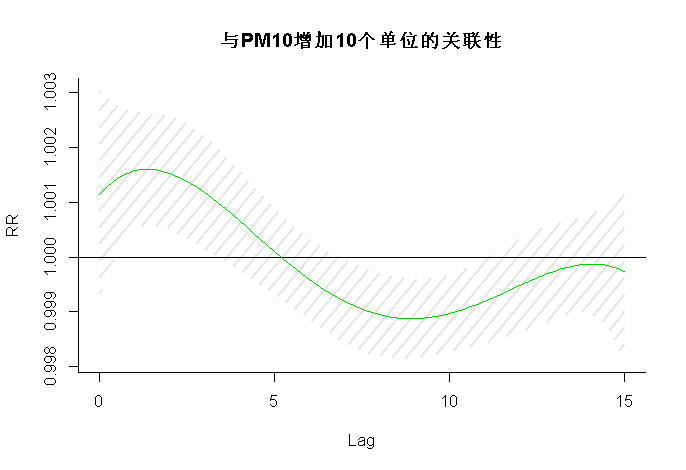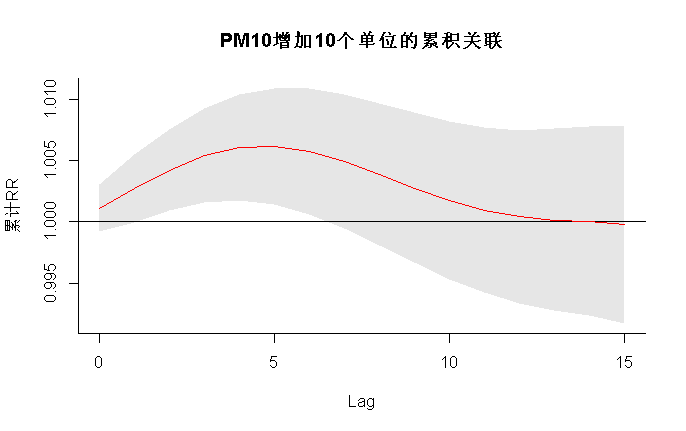PM10的特定值，即10 µgr / m3。使用0 µgr / m3的参考值定义此关联，从而为增加10个单位提供了特定于预测变量的关联。我还为第一个图选择了不同的颜色。参数cumul指示是否必须绘制以前保存在pred1.pm中的增量累积关联。结果如图1a-1b所示。置信区间被设置为参数ci的默认值“ area”。在左面板中，其他参数通过ci.arg传递给绘图函数polygon（），绘制阴影线作为置信区间。对这些曲线图的解释有两个方面：滞后曲线表示特定日期PM10增加10µgr/m3后未来每一天的风险增加（正向解释），或者过去每一天相同PM10对特定日期风险增加的贡献（反向解释）。图1a-1b中的曲线图表明，PM10风险的初始增加在较长的滞后时间被逆转。PM10在15天滞后时间内增加10个单位的总体累积效应（即将所有贡献相加至最大滞后时间）及其95%置信区间可通过pred1.pm中包含的对象allRRfit、allRRhigh和allRRlow提取，键入：

# R语言分布滞后线性和非线性模型（DLM和DLNM）建模

``````> pred1
10
0.9997563
> cbind(pred1.p
 0.9916871 1.0078911``````

# 例2：季节分析

``Sseas <- subset(NMMAPS, month %in% 6:9) ``

### 随时关注您喜欢的主题

``````cb(o3, lag=5,
argvar=list(fun="thr",thr=40.3), arglag=list(fun="integer"),
group=year) ``````

``````glm(death ~ cb2.o3 + cb2.temp + ns(doy, 4) + ns(time,3) + dow,
family=quasipoisson()) ``````

# 我绘制了O3增加10个单位的预测因子特定滞后反应关系，但置信区间为80％，并且还绘制了总体累积暴露反应关系。

``````> plot(pred2.o3, "slices", main="滞后响应 超过阈值10个单位"（80置信区间）)
> plot(pred2.o3,"overall",xlab="臭氧", ci="l", main="5个滞后的总体累积关联")``````

``````> pred2.o3\$allRRfit["50.3"]
50.3
1.047313``````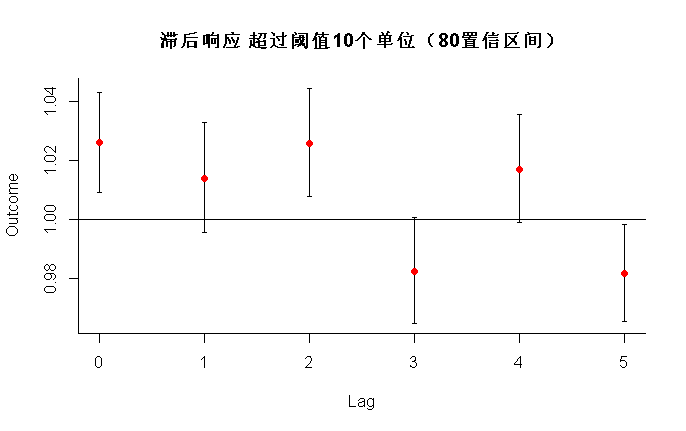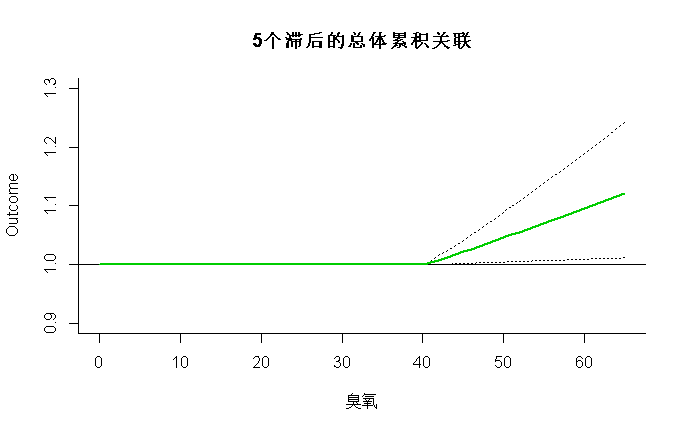``````> cbind(allRRlow, allRRhigh)["50.3",]
 1.004775 1.091652``````

# 示例3：二维DLNM

`````` > varknots <- equalknots(temp,fun="bs",df=5,degree=2)
> lagknots <- logknots(30, 3) ``````

`````` > plot(pred3.temp, xlab="温度" lphi=30,
main="温度效应的3D图")
> plot(pred3.temp, "contour", xlab="温度",
plot.title=title("等高线图",xlab="温度",ylab="滞后")) ``````

``````> plot(pred3.temp, "slices", var=-20,
main="不同温度下的滞后反应曲线，参考21C")
> for(i in 1:3) lines(pred3.temp, "slices", var=c(0,27,33)[i]
> legend("topright",paste("温度 =" ``````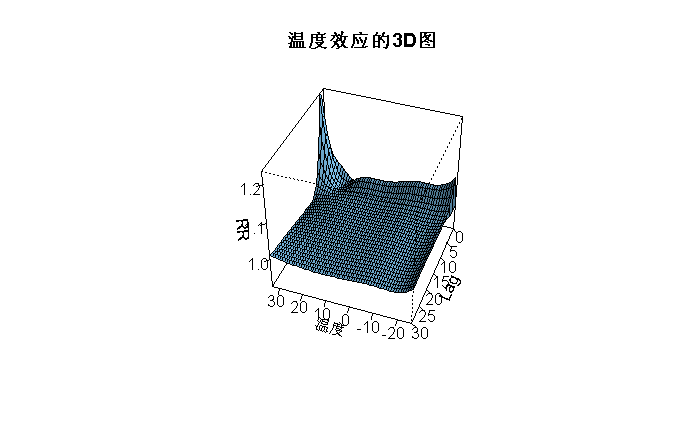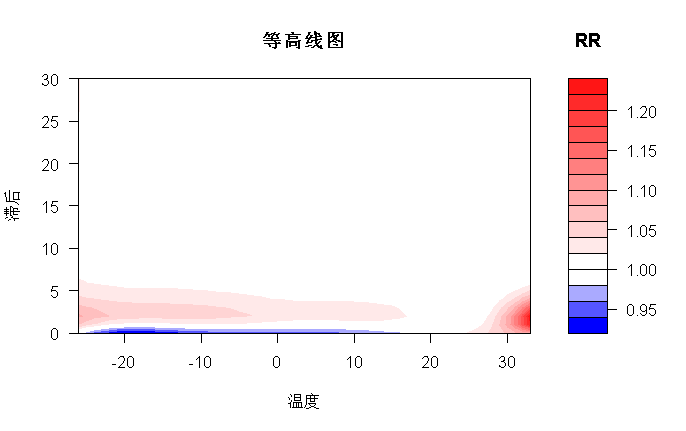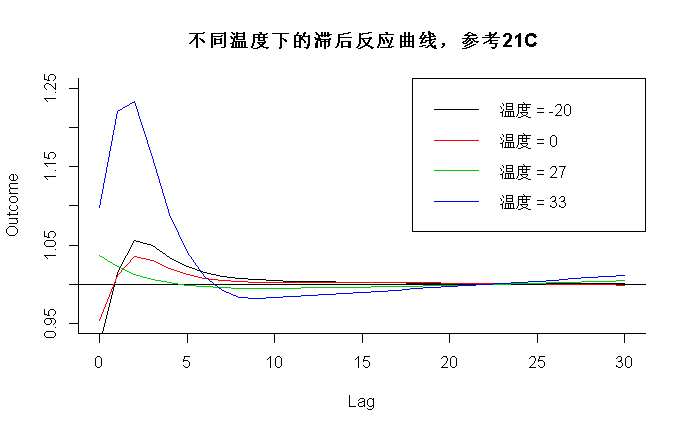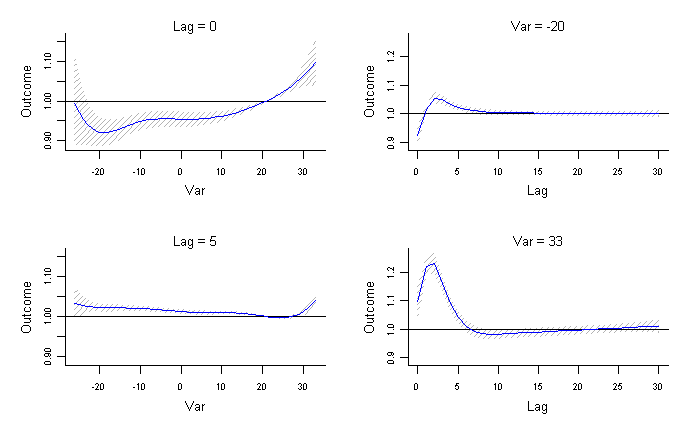# 示例4：降维DLNM

``credu(cb4, model4) ``

# 在最后一个例子中，我展示了如何使用函数crossreduce（）将二维DLNM的拟合度降低到由一维基的参数表示的摘要。首先，我指定一个新的交叉基矩阵，运行模型并以通常的方式进行预测

``````> length(coef(pred4))
 10
> length(coef(redall)) ; length(coef(redlag))
 2
 2
> length(coef(redvar))``````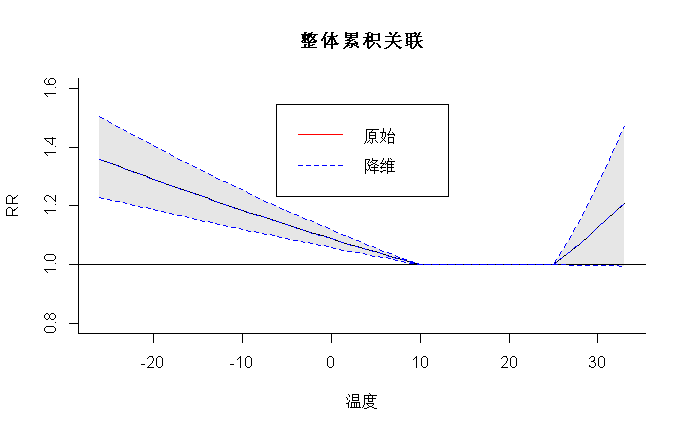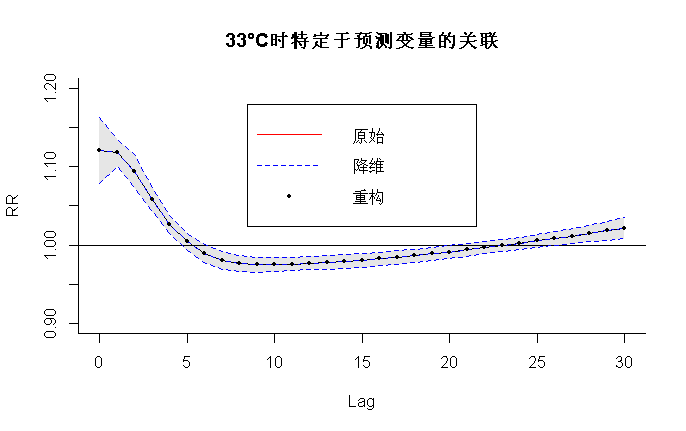``````> plot(pred4, "overall", xlab="温度", ylab="RR",
ylim=c(0.8,1.6), main="整体累积关联")
> lines(redall, ci="lines",col=4,lty=2)
> legend("top",c("原始","降维"),col=c(2,4),lty=1:2,ins=0.1) ``````

``````> plot(pred4, "slices", var=33,
main="33°C时特定于预测变量的关联")

> legend("top",c("原始","降维","重构"),``````

Kaizong Ye拓端研究室（TRL）的研究员。

​非常感谢您阅读本文，如需帮助请联系我们！QQ在线咨询

15121130882

0571-63341498

## 关注有关新文章的微信公众号

This will close in 0 seconds